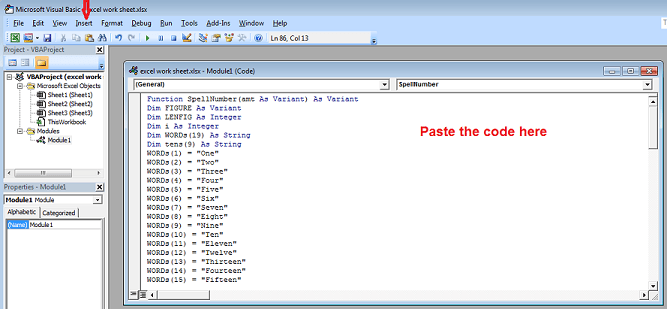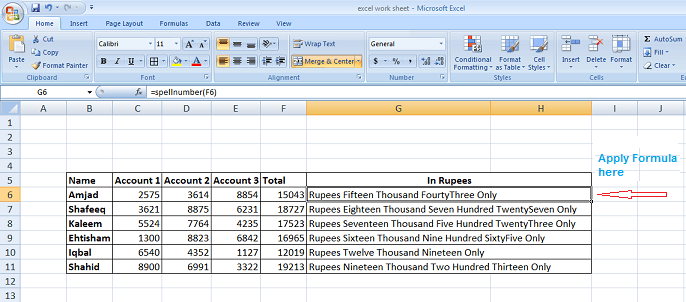# Convert Numbers into words in Excel in Indian and Pak Rupees

Most of the computer users know about Microsoft Excel and its importance in office work. Especially the ones who are linked with accounts and they often faced the situation where they have to convert numbers into words for the ease of their account holder and for other persons.

Let’s say we have a daily routine to manage some calculation and we have to manually input these results from numbers into words especially in Indian or Pakistan Rupees, then it must be annoying or boring to do the same practice and alternatively loss of valuable time. But need not to worry because I have compiled this article for such people who want to use this Excel function.

This trick will work for most of the Microsoft Excel versions (2003,2010,2013)

Don’t Miss:

## How to use SpellNumber Function to Convert Numbers into words in Excel in Indian and Pakistani Rupees:

It is very easy to convert numbers into words in Indian or Pakistan Rupees by using spellnumber function, just follow the steps given below.

STEP No.1:

copy the given code carefully or you can GET CODE FROM THIS LINK

```Function SpellNumber(amt As Variant) As Variant
Dim FIGURE As Variant
Dim LENFIG As Integer
Dim i As Integer
Dim WORDs(19) As String
Dim tens(9) As String
WORDs(1) = "One"
WORDs(2) = "Two"
WORDs(3) = "Three"
WORDs(4) = "Four"
WORDs(5) = "Five"
WORDs(6) = "Six"
WORDs(7) = "Seven"
WORDs(8) = "Eight"
WORDs(9) = "Nine"
WORDs(10) = "Ten"
WORDs(11) = "Eleven"
WORDs(12) = "Twelve"
WORDs(13) = "Thirteen"
WORDs(14) = "Fourteen"
WORDs(15) = "Fifteen"
WORDs(16) = "Sixteen"
WORDs(17) = "Seventeen"
WORDs(18) = "Eighteen"
WORDs(19) = "Nineteen"
tens(2) = "Twenty"
tens(3) = "Thirty"
tens(4) = "Fourty"
tens(5) = "Fifty"
tens(6) = "Sixty"
tens(7) = "Seventy"
tens(8) = "Eighty"
tens(9) = "Ninety"
FIGURE = amt
FIGURE = Format(FIGURE, "FIXED")
FIGLEN = Len(FIGURE)
If FIGLEN < 12 Then
FIGURE = Space(12 - FIGLEN) & FIGURE
End If
If Val(Left(FIGURE, 9)) > 1 Then
SpellNumber = "Rupees "
ElseIf Val(Left(FIGURE, 9)) = 1 Then
SpellNumber = "Rupee "
End If
For i = 1 To 3
If Val(Left(FIGURE, 2)) < 20 And Val(Left(FIGURE, 2)) > 0 Then
SpellNumber = SpellNumber & WORDs(Val(Left(FIGURE, 2)))
ElseIf Val(Left(FIGURE, 2)) > 19 Then
SpellNumber = SpellNumber & tens(Val(Left(FIGURE, 1)))
SpellNumber = SpellNumber & WORDs(Val(Right(Left(FIGURE, 2), 1)))
End If
If i = 1 And Val(Left(FIGURE, 2)) > 0 Then
SpellNumber = SpellNumber & " Crore "
ElseIf i = 2 And Val(Left(FIGURE, 2)) > 0 Then
SpellNumber = SpellNumber & " Lakh "
ElseIf i = 3 And Val(Left(FIGURE, 2)) > 0 Then
SpellNumber = SpellNumber & " Thousand "
End If
FIGURE = Mid(FIGURE, 3)
Next i
If Val(Left(FIGURE, 1)) > 0 Then
SpellNumber = SpellNumber & WORDs(Val(Left(FIGURE, 1))) + " Hundred "
End If
FIGURE = Mid(FIGURE, 2)
If Val(Left(FIGURE, 2)) < 20 And Val(Left(FIGURE, 2)) > 0 Then
SpellNumber = SpellNumber & WORDs(Val(Left(FIGURE, 2)))
ElseIf Val(Left(FIGURE, 2)) > 19 Then
SpellNumber = SpellNumber & tens(Val(Left(FIGURE, 1)))
SpellNumber = SpellNumber & WORDs(Val(Right(Left(FIGURE, 2), 1)))
End If
FIGURE = Mid(FIGURE, 4)
If Val(FIGURE) > 0 Then
SpellNumber = SpellNumber & " Paise "
If Val(Left(FIGURE, 2)) < 20 And Val(Left(FIGURE, 2)) > 0 Then
SpellNumber = SpellNumber & WORDs(Val(Left(FIGURE, 2)))
ElseIf Val(Left(FIGURE, 2)) > 19 Then
SpellNumber = SpellNumber & tens(Val(Left(FIGURE, 1)))
SpellNumber = SpellNumber & WORDs(Val(Right(Left(FIGURE, 2), 1)))
End If
End If
FIGURE = amt
FIGURE = Format(FIGURE, "FIXED")
If Val(FIGURE) > 0 Then
SpellNumber = SpellNumber & " Only "
End If
End Function
```

STEP No.2
Open the requires Excel Sheet and Press Alt+F11
STEP No.3
A new Windows will appear just click insert and then moduleSTEP No.4
Paste the code in box
STEP No.5
STEP No.6Keep the cursor in the box where you want to convert numbers into Words in Excel Sheet and type
=spellnumber(the cell number) i.e in the above if I want to convert F6 cell into words I will type =spellnumber(F6) and then enter

So in this way you can easily convert numbers into words in excel in Pakistani or Indian Rupees.

see the video tutorial for more guideline

### 23 thoughts on “Convert Numbers into words in Excel in Indian and Pak Rupees”

1.Thank you very much Sir
Sir
Excel main shops ya factory k hawaley sy koi data sheet / programme ho to plz btain

2.Great

3.Hi dear it is completely working just copy the code from the given link and carefully use it by following the steps mentioned.

4.Hi dear it is 100% working just copy the code from the given link and carefully use it by following the steps mentioned.

5.Dear

Thanks for the good work but need some adjustment like i have posted two outcomes of the spellnumber function,

Rupees One Thousand FiftyEight Only
Rupees Five Hundred NinetyNine Only

Can we also have a gap between fiftyeight and ninetynine.

6.Done Thanx Bro.

7.not working i m using ms excel 2007

•Hi dear it is 100% working just copy the code from the given link and carefully use it by following the steps mentioned.

8.HI
Dear please tel me how can i save this function permanently because when we re-open it, its not working . please tell me solution

9.I DON’T WANT RUPEES TO BE SHOWN JUST THE SPELL NUMBER

•Just remove Rupees from the above code.

•Hi thx for your interest…actually this excel function uses visual basics macros and script so it is not possible to save it permanently. You have to repeat the procedure every time to reopen the file.

10.Thanks Dear, Working very well

•you are welcome

11.hi friedns

it is working but when i am close same file and reopen again i need same practice again :( what should i do as i dont want to paste programming again and again.. guide me

•Hi, thanks for your comment, actually while implementing code, Excel is using its special function of “Macro” (It will record the application of formula and other data modification). So when you close and reopen it again, it will not work.
So kindly repeat the process to apply it again.

12.Great… Thank you for sharing such value able info …

13.Yes, Mr. Rafaqat,

Thanks for reply. But my query was unanswered. Is it required to do this copy and past job every time when a new excel file created? Because, I faced this situation. Please confirm.

•Hi dear since code is using macros so this copy paste job is required every time while using a new file.

14.Yes, Mr. Rafaqat, it is working. Earlier copy and paste was not done properly which I noticed now and rectified. But this process is cumbersome every time for everyfile we need to do this. Once copied it should work for all the existing and new files. Is n’t it?

•Yes it is working fine and hope so will work for every Microsoft Excel file….thx for visiting

15.•